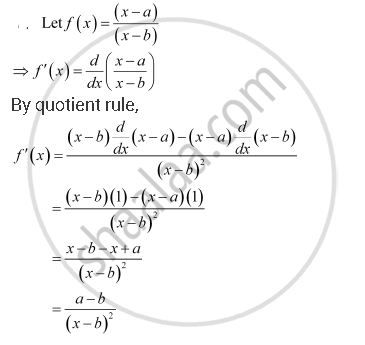Share

# For Some Constants A And B, Find the Derivative of (X-a)/(X -b) - Mathematics

#### Question

For some constants a and b, find the derivative of

(x-a)/(x -b)

#### SolutionIs there an error in this question or solution?

#### APPEARS IN

NCERT Solution for Mathematics Textbook for Class 11 (2018 (Latest))
Chapter 13: Limits and Derivatives
Q: 7.3 | Page no. 313

#### Video TutorialsVIEW ALL 

Solution For Some Constants A And B, Find the Derivative of (X-a)/(X -b) Concept: Derivative - Derivative of Polynomials and Trigonometric Functions.
S